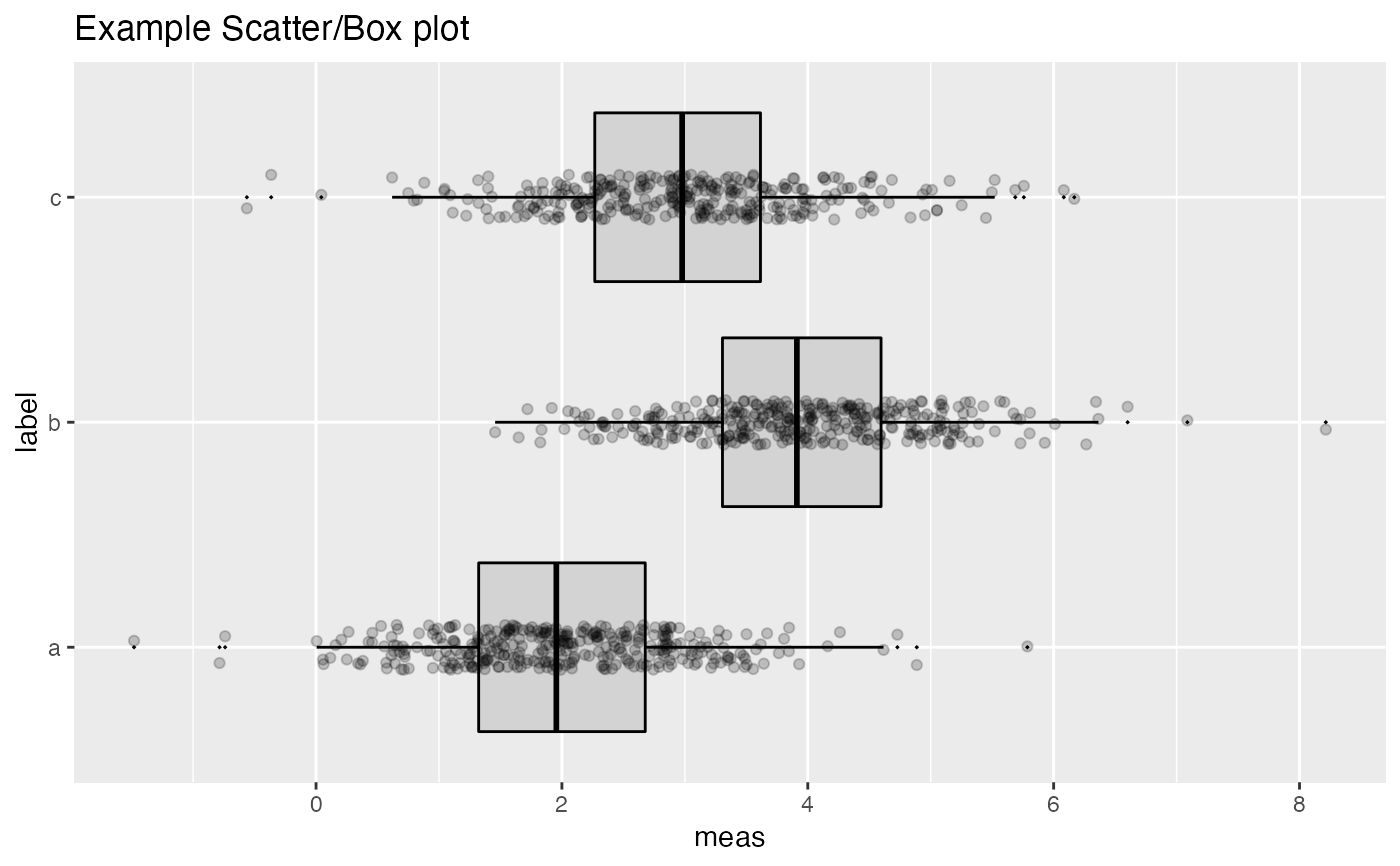Plot a boxplot with the data points superimposed. Box plots are aligned horizontally.

ScatterBoxPlotH(
frm,
xvar,
yvar,
title,
...,
pt_alpha = 0.3,
pt_color = "black",
box_color = "black",
box_fill = "lightgray"
)

## Arguments

frm data frame to get values from name of the continuous column in frame name of the independent column in frame; assumed discrete plot title (doesn't take additional arguments, used to force later arguments by name) transparency of points in scatter plot point color boxplot line color boxplot fill color (can be NA for no fill)

## Details

xvar is a continuous variable and yvar is a discrete variable.

ScatterBoxPlot

## Examples


classes = c("a", "b", "c")
means = c(2, 4, 3)
names(means) = classes
label = sample(classes, size=1000, replace=TRUE)
meas = means[label] + rnorm(1000)
frm2 = data.frame(label=label,
meas = meas)
WVPlots::ScatterBoxPlotH(frm2, "meas", "label",  pt_alpha=0.2, title="Example Scatter/Box plot")